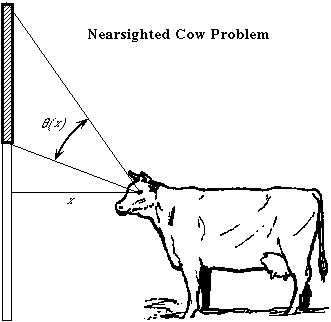The Nearsighted Cow Problem: A Calculus Classic.

A rectangular billboard 6 feet in height stands in a field so that its bottom is 10 feet above the ground. A nearsighted cow with eye level at 4 feet above the ground stands $x$ feet from the billboard. Express $\theta$, the vertical angle subtended by the billboard at her eye, in terms of $x$. Then find the distance $x_{0}$ the cow must stand from the billboard to maximize $\theta$.

$\theta(x)$ = $\theta$ is maximized when $x_{0}$ =( Click on image for a larger view )

You can earn partial credit on this problem.